Warning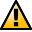This section not yet updated for Inkscape v0.92.

## Paths

Here is a listing for a path.

```
<path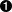style="fill:none;fill-opacity:0.7500000;fill-rule:evenodd;stro...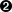d="M 174.0000,113.0000 L 221.0000,113.0000 L 221.0000,91.50000"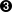id="path1370" />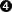```
 Start of Path object. Path style. (The line has been truncated.) Path commands. The possible path commands are given in the following list. An uppercase letter indicates that the coordinate(s) given are to be interpreted as absolute coordinates; a lowercase letter indicates they are to be interpreted relative to the last point. Parameters are given inside parentheses. Commands may be omitted in some circumstances. For example, "M 20 20 40 40" is to be interpreted as "M 20 20 L 40 40". For more details, see the SVG specification. M, m Move to (x, y). Move to a given point. Z, z Close path. Draw a straight line between last point and first point in path. The lines at the first point will be joined using the current Join style. L, l Line to (x, y). Draws a line from last point to new point. H, h Horizontal line to (x). Draws a line from last point to the point given by the new x coordinate (y coordinate is unchanged). V, v Vertical line to (y). Draws a line from last point to the point given by the new y coordinate (x coordinate is unchanged). C, c Curve to (x1, y1, x2, y2, x, y). Draws a Bezier curve from last point to the point given by the new x and y coordinates. x1 and y1 are coordinates of handle for old node; x2 and y2 are coordinates of handle for new node. S, s Smooth curve to (x2, y2, x, y). Draws a Bezier curve from last point to the point given by the new x and y coordinates. The coordinates of the handle for the old node, x1 and y1, are calculated assuming the old node is symmetric. x2 and y2 are coordinates of handle for new node. Q, q Quadratic curve to (x1, y1, x, y). Draws a Quadratic Bezier curve from last point to the point given by the new x and y coordinates. x1 and y1 are the coordinates of both the old and new node handles. T, t Symmetric Quadratic curve to (x, y). Draws a Quadratic Bezier curve from last point to the point given by the new x and y coordinates. The coordinates of the old and new handles are the same and are calculated assuming the old node is symmetric. A, a Elliptical Arc (rx, ry, x-axis-rotation, large-arc-flag sweep-flag, x, y). Draws an elliptical arc from the last point to the point given by x and y. See the SVG specification for more details. Path id and end of path definition.